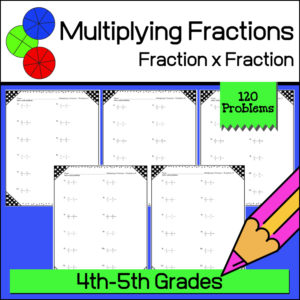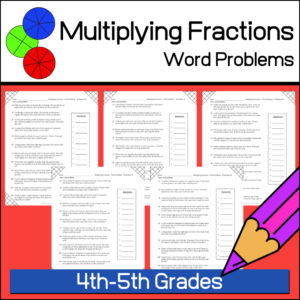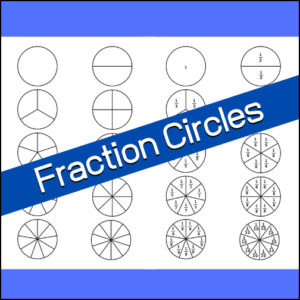Showing all 12 results

•\$3.00

Give your students the practice and reinforcement they need as they are learning to multiply fractions with fractions! This resource includes 120 problems (10 worksheets with 12 problems each) and answer keys.

•\$3.00

Help students develop their math word problem skills and practice solving multiplying fractions all at the same time! This resources provides ten (10) ready to use, print and go worksheets each with 12 word problems for a total of 120 problems (answer keys provided).

•\$2.50

Give your students the practice and reinforcement they need as they are learning to multiply fractions by whole numbers! This resource includes 100 problems (5 worksheets with 20 problems each) and answer keys.

•\$3.00

Help your students understand and remember the basis of multiplying fractions using a visual model method! This product, Multiplying Fractions with Visual Models #2, gives you 110 problems in which students will multiply a fraction with a another fraction. For each problem students will be asked to color in given visual fraction models to find the answer before giving the answer.

Product includes 10 worksheets (11 questions each) = 110 questions + answer keys.

•\$2.00

This resource will help students understand equivalent fractions, decimals and percents. It includes a poster / handout of explanation and a one page student worksheet. When given a fraction, decimal or percent, students will be tasked with providing the equivalent fraction, decimal or percent. Answer Key provided.

•\$2.50

4 Christmas themed Math worksheets for students to practice changing fractions into decimals.

• Worksheet #1
Fractions with denominators of 2, 4, 5 and 10
• Worksheet #2
Fractions with denominators of 3, 5, 6, 7, 9 and 10
• Worksheet #3
Fractions with denominators of 10, 100 and 1000
(Highest fraction 694/1000)
• Worksheet #4
Fractions with denominators of 10, 100 and 1000
(Will include mixed fractions – example: 3337/1000)

•\$3.00

15 Math worksheets for students to practice and show their understanding of equivalent fractions! Answer Keys provided

Resource includes:

• 10 worksheets requiring students to reduce a fraction to the lowest equivalent fraction
• 3 worksheets asking students to choose the equivalent fraction from a given set
• 2 worksheets where students are to complete a pattern by writing in the missing equivalent fraction.
•\$3.00

15 Christmas themed Math worksheets for students to practice and show their understanding of equivalent fractions! Answer Keys provided

Resource includes:

• 10 worksheets requiring students to reduce a fraction to the lowest equivalent fraction
• 3 worksheets asking students to choose the equivalent fraction from a given set
• 2 worksheets where students are to complete a pattern by writing in the missing equivalent fraction.
•\$3.00

Help your students understand and remember the basis of multiplying fractions using a visual model method! This product gives you 120 problems in which students will multiply a fraction with a whole number. For each problem students will be asked to color in given visual fraction models to find the answer. Then they are to write the answers using mixed numbers.

Product includes 10 worksheets (12 questions each) = 120 questions + answer keys.

•\$2.00

4 Fraction Wall posters (or handouts)! You’ll get 2 in color (one with fractions and one without) and 2 in b/w (also one with fractions and one without.

•\$3.00

Help your students reinforce their knowledge and Math skills with these 15 worksheets (210 problems) on dividing fractions! Students will divide fractions (numerator of 1) by whole numbers and whole numbers by fractions (numerator of 1).

•\$1.25

Here is a 2 page resource for students to use when learning about fractions. Page one shows fraction circles with no labels. Page two has the identical fraction circles only they are labeled. (All B/W)

Fractions: 1 whole, 1/2, 1/3, 1/4, 1/5, 1/6, 1/7, 1/8, 1/9, 1/10